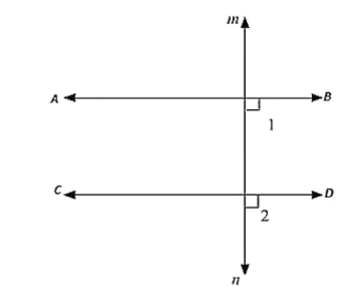# If each of the two lines is perpendicular to the same line, what kind of lines are they to each other?

Question:

If each of the two lines is perpendicular to the same line, what kind of lines are they to each other?3Solution:

Let AB and CD be perpendicular to MN

ABD = 90 [AB perpendicular to MN]   ..... (i)

CON = 90 [CO perpendicular to MN]    ....  (ii)

Now, ABD = CDN = 90 (From (i) and (ii))

AB parallel to CD,

Since corresponding angles are equal